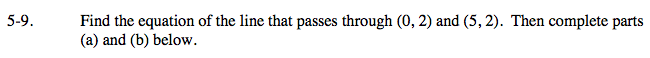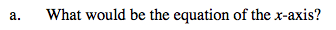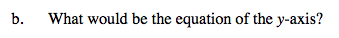### Home > A2C > Chapter 5 > Lesson 5.1.1 > Problem5-9

5-9.
1. Find the equation of the line that passes through (0, 2) and (5, 2). Then complete parts (a) and (b) below. Homework Help ✎

1. What would be the equation of the x-axis?

2. What would be the equation of the y-axis?What points must the x-axis pass through?What points must the y-axis pass through?